# Implementing a BST where every node stores the maximum number of nodes in the path till any leaf

Given an array of values. The task is to implement a Binary Search Tree using values of the array where every node stores the maximum number of nodes in the path starting from the node itself and ending at any leaf of the tree.

Note: The maximum number of nodes in the path from any node to any leaf node in a BST is the height of the subtree rooted at that node.

Examples:

Input : arr[] = {1, 2, 3, 4, 5, 6, 7}
Output :
data = 1 height = 6
data = 2 height = 5
data = 3 height = 4
data = 4 height = 3
data = 5 height = 2
data = 6 height = 1
data = 7 height = 0

Input : arr[] = {4, 12, 10, 5, 11, 8, 7, 6, 9}
Output :
data = 4 height = 6
data = 5 height = 3
data = 6 height = 0
data = 7 height = 1
data = 8 height = 2
data = 9 height = 0
data = 10 height = 4
data = 11 height = 0
data = 12 height = 5

## Recommended: Please try your approach on {IDE} first, before moving on to the solution.

The idea is to add nodes in the BST fashion. Height of the parent say P will be updated only when the new node is added to the subtree which contributes to the height of P AND (logical) the height of the subtree has increased as well after the addition of the new node.

Let’s say that an existing tree is (data of node is in red and current height of node in green):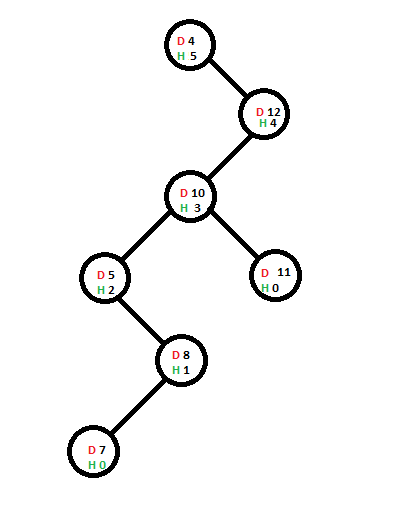Now we are going to add a new node of containing the value 6, the route taken by node in order to get added has been highlighted in blue: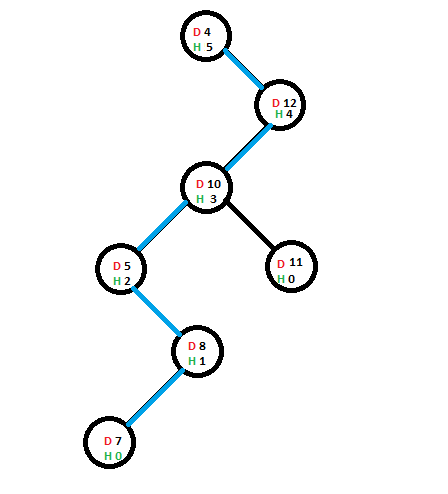With addition of the new node, the height of it’s immediate parent will be increased (only if the height of the immediate parent of node containing 6 is being affected by this addition – which in this case is true). Once the height of parent is incremented, it will check whether the sub-tree where parent is present is the main contributory to the height of node having that sub-tree as a child, if yes then height of that node will be increased – in short the height incrementation if propagated upwards.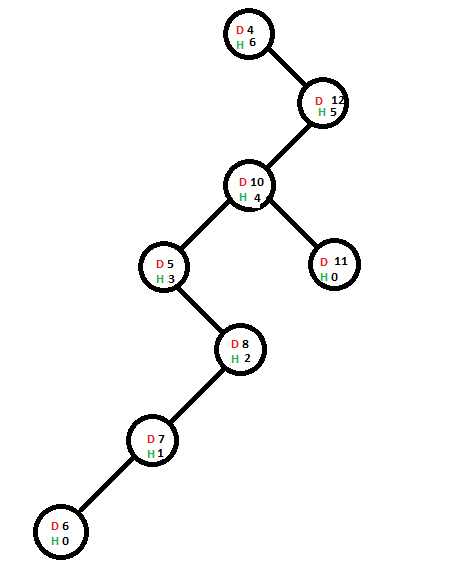Now we are going to add another node containing value 9 & the path it will take to get added to its final position is in blue: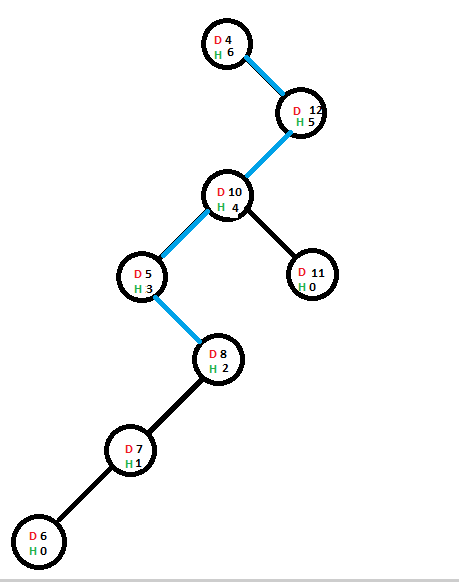Since the height of the immediate parent of the node containing value 9 is not getting affected by this addition, its parent’s height won’t get affected and height incrementation won’t get propagated upwards.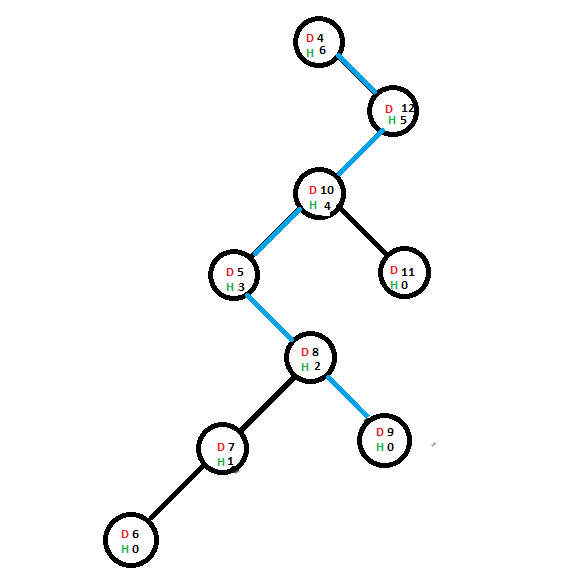Below is the implementation of the above approach:

## C

 `// C implementation of above approach ` `#include ` `#include ` ` `  `// Structure containing basic template od a node ` `struct` `node { ` ` `  `    ``// Stores the data and current height of the node ` `    ``int` `data; ` `    ``int` `height; ` `    ``struct` `node* right; ` `    ``struct` `node* left; ` `}; ` ` `  `int` `indicator = 0; ` `void` `left_insert(``struct` `node*, ``struct` `node*); ` `void` `right_insert(``struct` `node*, ``struct` `node*); ` ` `  `// Inorder traversal of the tree ` `void` `traverse(``struct` `node* head) ` `{ ` `    ``if` `(head != NULL) { ` `        ``traverse(head->left); ` `        ``printf``(``" data   = %d"``, head->data); ` `        ``printf``(``" height = %d\n"``, head->height); ` `        ``traverse(head->right); ` `    ``} ` `} ` ` `  `// Insertion to the left sub-tree ` `void` `left_insert(``struct` `node* head, ``struct` `node* temp) ` `{ ` `    ``// Child node of Current head ` `    ``struct` `node* child = NULL; ` ` `  `    ``if` `(head->data > temp->data) { ` `        ``if` `(head->left == NULL) { ` `            ``indicator = 1; ` `            ``child = head->left = temp; ` `        ``} ` `        ``else` `{ ` `            ``left_insert(head->left, temp); ` `            ``child = head->left; ` `        ``} ` `    ``} ` `    ``else` `{ ` `        ``right_insert(head, temp); ` `    ``} ` ` `  `    ``if` `((indicator == 1) && (child != NULL)) { ` `        ``if` `(head->height > child->height) { ` `            ``// Ending propagation to height of above nodes ` `            ``indicator = 0; ` `        ``} ` `        ``else` `{ ` `            ``head->height += 1; ` `        ``} ` `    ``} ` `} ` ` `  `// Insertion to the right sub-tree ` `void` `right_insert(``struct` `node* head, ``struct` `node* temp) ` `{ ` `    ``// Child node of Current head ` `    ``struct` `node* child = NULL; ` ` `  `    ``if` `(head->data < temp->data) { ` `        ``if` `(head->right == NULL) { ` `            ``indicator = 1; ` `            ``child = head->right = temp; ` `        ``} ` `        ``else` `{ ` `            ``right_insert(head->right, temp); ` `            ``child = head->right; ` `        ``} ` `    ``} ` `    ``else` `{ ` `        ``left_insert(head, temp); ` `    ``} ` ` `  `    ``if` `((indicator == 1) && (child != NULL)) { ` `        ``if` `(head->height > child->height) { ` ` `  `            ``// Ending propagation to height of above nodes ` `            ``indicator = 0; ` `        ``} ` `        ``else` `{ ` `            ``head->height += 1; ` `        ``} ` `    ``} ` `} ` ` `  `// Function to create node and push ` `// it to its appropriate position ` `void` `add_nodes(``struct` `node** head, ``int` `value) ` `{ ` `    ``struct` `node *temp_head = *head, *temp; ` ` `  `    ``if` `(*head == NULL) { ` `        ``// When first node is added ` `        ``*head = ``malloc``(``sizeof``(**head)); ` `        ``(*head)->data = value; ` `        ``(*head)->height = 0; ` `        ``(*head)->right = (*head)->left = NULL; ` `    ``} ` `    ``else` `{ ` `        ``temp = ``malloc``(``sizeof``(*temp)); ` `        ``temp->data = value; ` `        ``temp->height = 0; ` `        ``temp->right = temp->left = NULL; ` `        ``left_insert(temp_head, temp); ` `        ``temp_head = *head; ` `        ``indicator = 0; ` `    ``} ` `} ` ` `  `// Driver Code ` `int` `main() ` `{ ` `    ``struct` `node *head = NULL, *temp_head = NULL; ` ` `  `    ``add_nodes(&head, 4); ` `    ``add_nodes(&head, 12); ` `    ``add_nodes(&head, 10); ` `    ``add_nodes(&head, 5); ` `    ``add_nodes(&head, 11); ` `    ``add_nodes(&head, 8); ` `    ``add_nodes(&head, 7); ` `    ``add_nodes(&head, 6); ` `    ``add_nodes(&head, 9); ` ` `  `    ``temp_head = head; ` ` `  `    ``// Traversing the tree to display  ` `    ``// the updated height values ` `    ``traverse(temp_head); ` `    ``return` `0; ` `} `

## Java

 `// Java implementation of above approach ` `class` `GFG ` `{ ` ` `  `// Structure containing basic template od a node ` `static` `class` `node  ` `{ ` ` `  `    ``// Stores the data and current height of the node ` `    ``int` `data; ` `    ``int` `height; ` `    ``node right; ` `    ``node left; ` `} ` ` `  `static` `int` `indicator = ``0``; ` ` `  `// Inorder traversal of the tree ` `static` `void` `traverse(node head) ` `{ ` `    ``if` `(head != ``null``) ` `    ``{ ` `        ``traverse(head.left); ` `        ``System.out.printf(``" data = %d"``, head.data); ` `        ``System.out.printf(``" height = %d\n"``, head.height); ` `        ``traverse(head.right); ` `    ``} ` `} ` ` `  `// Insertion to the left sub-tree ` `static` `void` `left_insert(node head, node temp) ` `{ ` `    ``// Child node of Current head ` `    ``node child = ``null``; ` ` `  `    ``if` `(head.data > temp.data) ` `    ``{ ` `        ``if` `(head.left == ``null``) ` `        ``{ ` `            ``indicator = ``1``; ` `            ``child = head.left = temp; ` `        ``} ` `        ``else`  `        ``{ ` `            ``left_insert(head.left, temp); ` `            ``child = head.left; ` `        ``} ` `    ``} ` `    ``else` `    ``{ ` `        ``right_insert(head, temp); ` `    ``} ` ` `  `    ``if` `((indicator == ``1``) && (child != ``null``)) ` `    ``{ ` `        ``if` `(head.height > child.height) ` `        ``{ ` `             `  `            ``// Ending propagation to height of above nodes ` `            ``indicator = ``0``; ` `        ``} ` `        ``else` `        ``{ ` `            ``head.height += ``1``; ` `        ``} ` `    ``} ` `} ` ` `  `// Insertion to the right sub-tree ` `static` `void` `right_insert(node head, node temp) ` `{ ` `    ``// Child node of Current head ` `    ``node child = ``null``; ` ` `  `    ``if` `(head.data < temp.data)  ` `    ``{ ` `        ``if` `(head.right == ``null``) ` `        ``{ ` `            ``indicator = ``1``; ` `            ``child = head.right = temp; ` `        ``} ` `        ``else`  `        ``{ ` `            ``right_insert(head.right, temp); ` `            ``child = head.right; ` `        ``} ` `    ``} ` `    ``else` `    ``{ ` `        ``left_insert(head, temp); ` `    ``} ` ` `  `    ``if` `((indicator == ``1``) && (child != ``null``))  ` `    ``{ ` `        ``if` `(head.height > child.height) ` `        ``{ ` ` `  `            ``// Ending propagation to height of above nodes ` `            ``indicator = ``0``; ` `        ``} ` `        ``else`  `        ``{ ` `            ``head.height += ``1``; ` `        ``} ` `    ``} ` `} ` ` `  `// Function to create node and push ` `// it to its appropriate position ` `static` `node add_nodes(node head, ``int` `value) ` `{ ` `    ``node temp_head = head, temp; ` ` `  `    ``if` `(head == ``null``)  ` `    ``{ ` `        ``// When first node is added ` `        ``head = ``new` `node(); ` `        ``(head).data = value; ` `        ``(head).height = ``0``; ` `        ``(head).right = (head).left = ``null``; ` `    ``} ` `    ``else` `    ``{ ` `        ``temp = ``new` `node(); ` `        ``temp.data = value; ` `        ``temp.height = ``0``; ` `        ``temp.right = temp.left = ``null``; ` `        ``left_insert(temp_head, temp); ` `        ``temp_head = head; ` `        ``indicator = ``0``; ` `    ``} ` `    ``return` `head; ` `} ` ` `  `// Driver Code ` `public` `static` `void` `main(String args[]) ` `{ ` `    ``node head = ``null``, temp_head = ``null``; ` ` `  `    ``head = add_nodes(head, ``4``); ` `    ``head = add_nodes(head, ``12``); ` `    ``head = add_nodes(head, ``10``); ` `    ``head = add_nodes(head, ``5``); ` `    ``head = add_nodes(head, ``11``); ` `    ``head = add_nodes(head, ``8``); ` `    ``head = add_nodes(head, ``7``); ` `    ``head = add_nodes(head, ``6``); ` `    ``head = add_nodes(head, ``9``); ` ` `  `    ``temp_head = head; ` ` `  `    ``// Traversing the tree to display  ` `    ``// the updated height values ` `    ``traverse(temp_head); ` `} ` `} ` ` `  `// This code is contributed by Arnab Kundu `

Output:

``` data   = 4 height = 6
data   = 5 height = 3
data   = 6 height = 0
data   = 7 height = 1
data   = 8 height = 2
data   = 9 height = 0
data   = 10 height = 4
data   = 11 height = 0
data   = 12 height = 5
```

Attention reader! Don’t stop learning now. Get hold of all the important DSA concepts with the DSA Self Paced Course at a student-friendly price and become industry ready.

My Personal Notes arrow_drop_upCheck out this Author's contributed articles.

If you like GeeksforGeeks and would like to contribute, you can also write an article using contribute.geeksforgeeks.org or mail your article to contribute@geeksforgeeks.org. See your article appearing on the GeeksforGeeks main page and help other Geeks.

Please Improve this article if you find anything incorrect by clicking on the "Improve Article" button below.

Improved By : andrew1234